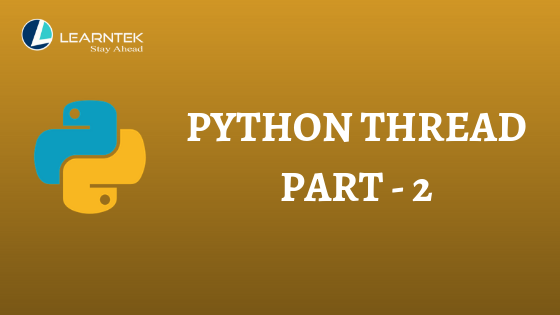# Python Threading – Part 2

So far, we have created the non-daemon thread. What is the daemon thread? When the main thread exits, it attempts to terminate all of its daemonic child threads.
Consider an example of GUI as shown below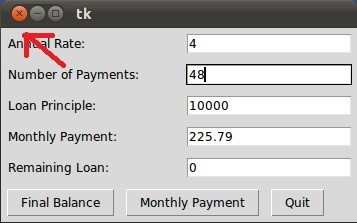Consider, by GUI input some calculation is being performed in the background and the calculation is taking its time.

If you click close button two courses of action can be performed.

1. After clicking the close button the whole GUI window exists.
2. After clicking the close button the GUI window will wait for the completion of background calculation.

If the first course of action is performed then Daemon thread are being used in background calculation. If the second course of action is performed then a Non-daemon thread is being used in background calculation.

Let us understand with the help of code

```import threading

import time

def n():

print ("Non deamon start")

print ("NOn daemoon exit")

def d():

print (" daemon start")

time.sleep(5)

print (" daemon stop")

d.setDaemon(True)

d.start()

t.start()```

If method isDaemon() returns True then the thread is a daemon thread. The syntax d.setDaemon(True) or d.daemon = True can be used to make daemon thread.

Let us see the output: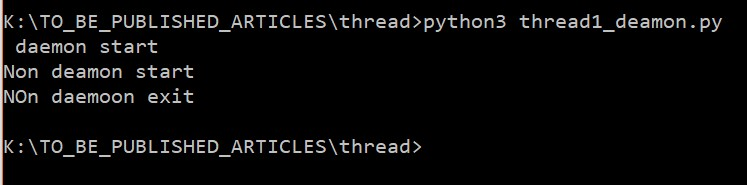Daemon thread will take 5 seconds to complete its task, but main did not wait for the daemon thread. That’s why in the output there is no “daemon stop” statement. Now remove the time.sleep(5) from function d() and add it in n() function.

See the code below.

```import threading

import time

def n():

print ("Non deamon start")

time.sleep(5)

print ("NOn daemoon exit")

def d():

print (" daemon start")

print (" daemon stop")

d.setDaemon(True)

d.start()

t.start()```

See the output: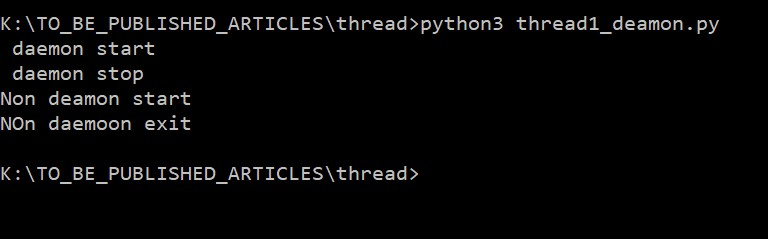In the above example, all print statements are executed. The main thread had to wait for the non-daemon process.

Note: If you use join statement for Daemon thread then the main thread has to wait for the completion of Daemon thread’s task.

Learn Python + Advanced Python with our Experts

#### Locks

Locks are the most fundamental synchronization mechanism provided by the threading module. A lock is in one of two states, locked or unlocked. If a thread attempts to hold a lock that’s already held by some other thread, execution of the second thread is halted until the lock is released.

lock.acquire ():
Acquire a lock, blocks others until True (Default )

lock.locked():
Returns True if lock is locked, otherwise False.

lock.release():
Unlocks the lock

Let us see one example.

```import threading

import time

list1 = []

def fun1(a):

lock.acquire()

list1.append(a)

lock.release()

for each in range(10):

print ("List1 is : ", list1)```

The lock = threading.Lock() is used to create a lock object.

The main problem with the lock is, the lock does not remember which thread acquired the lock. Now two problem can be aroused.

See the code below.

```import threading

import time

import datetime

t1 = datetime.datetime.now()

def second(n):

lock.acquire()

print (n)

def third():

time.sleep(5)

lock.release()

th1.start()

th2.start()

th3.start()

th1.join()

th2.join()

th3.join()

t2 = datetime.datetime.now()

print ("Total time", t2-t1)```

In the above code, a lock is acquired by thread1 and released by thread3. The thread2 is trying to acquire the lock.
Let us see the output.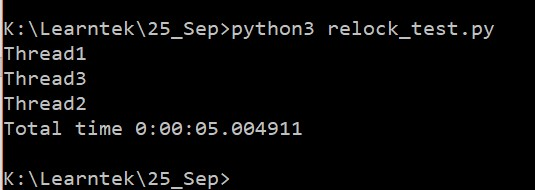From the sequence of execution, it is clear that the lock acquired by the thread1 got released by the thread3.

Let see second problem.

```import threading

def first(n):

lock.acquire()

a =12+n

lock.release()

print (a)

def second(n):

lock.acquire()

b = 12+n

lock.release()

print (b)

def all():

lock.acquire()

first(2)

second(3)

lock.release()

th1.start()```

When you run the above code, deadlock would occur. In the function all thread will acquire a lock, after acquiring the lock the first function will be called. The thread will see the lock.acquire() statement. As this lock itself acquired by the same thread. But lock does not remember the thread which acquired it.

In order to overcome above problem, we use Reentrant lock (RLock).

threading.RLock() — A factory function that returns a new reentrant lock object. A reentrant lock must be released by the thread that acquired it. Once a thread has acquired a reentrant lock, the same thread may acquire it again without blocking; the thread must release it once for each time it has acquired it.

Lock vs Rlock

The main difference is that a Lock can only be acquired once. It cannot be acquired again until it is released. (After it’s been released, it can be re-acquired by any thread).

An RLock, on the other hand, can be acquired multiple times, by the same thread. It needs to be released the same number of times in order to be “unlocked”.

Another difference is that an acquired Lock can be released by any thread, while an acquired RLock can only be released by the thread which acquired it.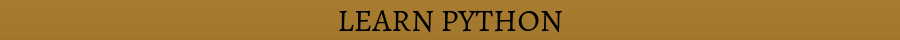GIL

Thread-based parallelism is the standard way of writing parallel programs. However, the Python interpreter is not fully thread-safe. In order to support multi-threaded Python programs, a global lock called the Global Interpreter Lock (GIL) is used. This means that only one thread can execute the Python code at the same time; Python automatically switches to the next thread after a short period of time or when a thread does something that may take a while. The GIL is not enough to avoid problems in your own programs. Although, if multiple threads attempt to access the same data object, it may end up in an inconsistent state.

Let us see the example.

```import datetime

def count(n):

t1 = datetime.datetime.now()

while n > 0:

n = n-1

t2 = datetime.datetime.now()

print (t2-t1)

count(100000000)```

In the above code, the count function is being run the main thread. Let see the time taken by the thread.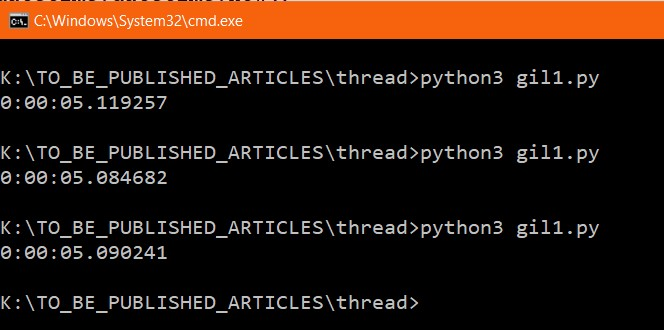I ran the code three times, every time I got a similar result.

Let us create two thread, see the code below.

```import datetime

def count(n):

while n > 0:

n = n-1

def count1(n):

while n > 0:

n = n-1

t1 = datetime.datetime.now()

t2 = datetime.datetime.now()

print (t2-t1)```

In the above, two threads have been created to be run in parallel.

Let us see the result.You can the above code took almost 10 seconds which is the double of the previous program, it means, only the main thread act as multithreading. But above experiment, we can conclude that Multithreading is defined as the ability of a processor to execute multiple threads concurrently.

In a simple, single-core CPU, it is achieved using frequent switching between threads. This is termed as context switching.
In context switching, the state of a thread is saved and state of another thread is loaded whenever any interrupt (due to I/O or manually set) takes place. Context switching takes place so frequently that all the threads appear to be running parallelly(this is termed as multitasking)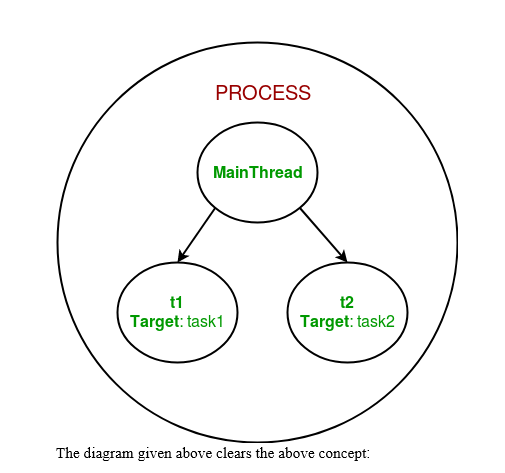I hope you enjoyed the article. 😊